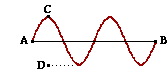Waves, Sound and Light: Wave Basics

Wave Basics: Audio Guided Solution

Problem 17:A wave with a frequency of 12.3 Hz is traveling from left to right across a rope as shown in the diagram at the right Positions A and B in the diagram are separated by a horizontal distance of 42.8 cm. Positions C and D in the diagram are separated by a vertical distance of 12.4 cm. Determine the amplitude, wavelength, period and speed of this wave.

Audio Guided Solution

Click to show or hide the answer!

Habits of an Effective Problem Solver

• Read the problem carefully and develop a mental picture of the physical situation. If necessary, sketch a simple diagram of the physical situation to help you visualize it.
• Identify the known and unknown quantities and record in an organized manner, often times they can be recorded on the diagram itself. Equate given values to the symbols used to represent the corresponding quantity (e.g., v = 12.8 m/s, λ = 4.52 m, f = ???).
• Use physics formulas and conceptual reasoning to plot a strategy for solving for the unknown quantity.
• Identify the appropriate formula(s) to use.
• Perform substitutions and algebraic manipulations in order to solve for the unknown quantity.